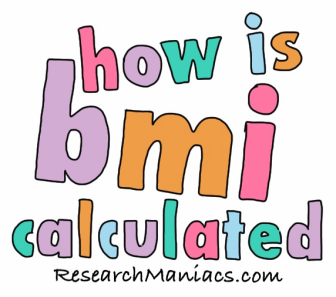How is BMI calculated?

Question: How is BMI calculated?

The BMI was first used in the 1800s when it was called the Quetelet Index. It was not until the 1970s that it is was referred to as BMI or the Body Mass Index.

Basically, the BMI is used to measure body fat by looking at the ratio of weight to height.

To calculate BMI, you can either use pounds and inches, or kilos and meters. The two alternative formulas for calculating BMI are as follows:

BMI = (Pound / Inches^2) x 703

BMI = Kilo / Meters^2

Now you know how BMI is calculated, check out other Interesting information below about weight and BMI.

 How Is? How is this, how is that? Find out here! How to Lose Weight This is how you lose weight for free. Research Maniacs' 4-step weight loss program. How many calories should I eat? Calculate how many calories you should eat? iPhone/iPad BMI App This App will first calculate your BMI, and then explain and suggest what your BMI should be. How much should I weigh? How much should I weighfor my height? What is my BMI? Are you overweight? Calculate your BMI here!